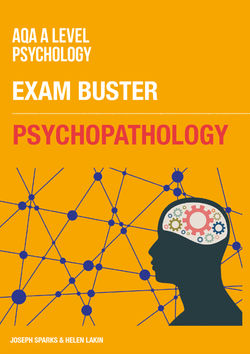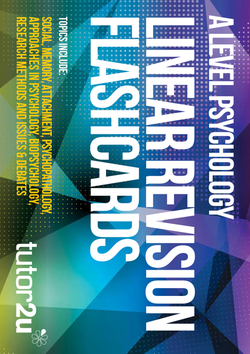# Psychology

Explore PsychologySearch
Study notes

# Measures of Central Tendency

• Levels: AS, A Level
• Exam boards: AQA, Edexcel, OCR, IB

Descriptive statistics are analyses of quantitative [numerical] data that summarise patterns, and therefore often save readers from trawling through large amounts of data to understand research findings.

Measures of central tendency are examples of descriptive data statistics that depict an overall ‘central’ trend of a set of data. There are three key measures:

Mode – the most frequently occurring number in a data set.

e.g. the mode of 1, 2, 3, 4, 4, 5 is 4

Median – the middle score when the data are in numerical order.

e.g. the median of numbers 1, 2, 3, 4, 5 would be 3),

If there are an even number of data then this is the sum of the two middle numbers, divided by two (e.g. the median of 1, 2, 3, 4, would be [2+3] / 2 = 2.5)

Mean – sometimes referred to as the average, stemming from the method to calculate it: the sum of all numbers in the data set, divided by how many numbers there are in the data set.

e.g. the mean of 1, 2, 3, 4, 5, 6 would be calculated:

[1+2+3+4+5+6] / 6 = 3.5

The mean takes all numbers of a data set into account, which could be deemed as a strength of the measure, but this also means that it is susceptible to skewing of the final calculated figure if the data features extreme values.

For example, in a data set – 1, 2, 3, 4, 19 – the mean would be 5.8

i.e. [1+2+3+4+19] / 2)

which could be argued as unrepresentative as most values in the data set are smaller than 5.8.

From this perspective, the median (i.e. 3) might be a better descriptive statistic to report, as it yields a value that is unaffected by any extreme values.

The mode can be useful by showing the most frequent value(s) in a data set, but it is of little use where the data set includes many different values of the same frequency, i.e. there are many modes.

For example, in a data set –1, 2, 3, 4, 19 – all 5 values are the mode, which does not summarise the data at all.

• 36

Collections

• ### Research Methods: MCQ Revision Test 1 for AQA A Level Psychology

Student videos

• ### Research Methods - Descriptive Statistics

Revision quizzes
• ### A Level Psychology Topic Quiz - Research Methods

Revision quizzes

Study notes

Study notes

Study notes

Study notes

Study notes

Study notes

Study notes

Study notes

Study notes
• ### Relationships: Virtual Relationships

Study notes
• All Study notes ›## Psychopathology: Exam Buster Revision Guide for AQA A Level Psychology

• SKU: 03-4130-30107-03
• Printed Edition

• £5.95## Core Topics Revision Flashcards for AQA A Level Psychology

• SKU: 03-4130-30001-03
• Printed Edition

• £7.95 (+VAT)

Recruitment